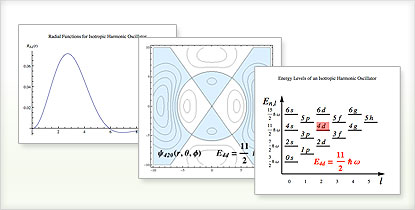# The Wolfram Solution for Chemistry

The Wolfram chemistry solution offers a complete suite of tools for analytical, physical, organic, and inorganic chemistry, including high-powered data analysis, interactive visualization and automatic reporting—all in one system.

Curated chemical and scientific data is built into Mathematica alongside highly automated computation for ease and accuracy of calculations.

• The Wolfram Edge
• How Wolfram Compares
• Key Capabilities

Wolfram technologies include thousands of built-in functions and curated data on many topics that let you:

• Access physical and safety properties of chemicals in your laboratory using built-in chemical data
• Calculate path-dependent and path-independent quantities such as entropy, free energy, chemical potential and more
• Simulate mass transport and chemical kinetics such as electrochemical reactions
• Calculate the time-independent Schrödinger equation and its solutions in terms of wavefunctions and their eigenvalues, and other applications in quantum chemistry
• Solve coupled nonlinear differential equations for chemical kinetics modeling
• Interactively visualize molecular structures of biochemical compoundsGenerating the solution of a wave function and its corresponding energy for an isotropic 3D harmonic oscillatorIllustrating the chirality of substituted methanes

• Built-in chemical, element, lattice and isotope data, ready for use without preprocessing
Unique to Wolfram technologies
• Integrated automatic report generation to document tasks you perform in Mathematica and instantly generate reports with live graphics, text, and executable code
• Import or acquire data, perform statistical analyses and visualize results in one system instead of across several applications
• Highly optimized superfunctions with automated algorithm selection to get accurate results quickly—sometimes switching algorithms mid-calculation for further optimization
Non-Wolfram computation systems make you analyze your equations manually to determine which function to apply—for example, where in the Wolfram Language you use NDSolve, in Matlab you must correctly choose among obscure named algorithms like ode45, ode23, ode113, ode15s, bvp4c, pdepe and so on, or risk wrong answers
• Create interactive tools for designing chemical instrumentation, curve fitting or data analysis that provide visual feedback to make debugging and testing of innovative instrumentation easier
Unique to Wolfram technologies
• Integrated environment for chemical kinetic modeling, statistical analysis, optimization and generation of interactive reports and applications
• Accurate solving of highly nonlinear problems in transport phenomena and other areas with built-in arbitrary-precision numerics and automatic precision control